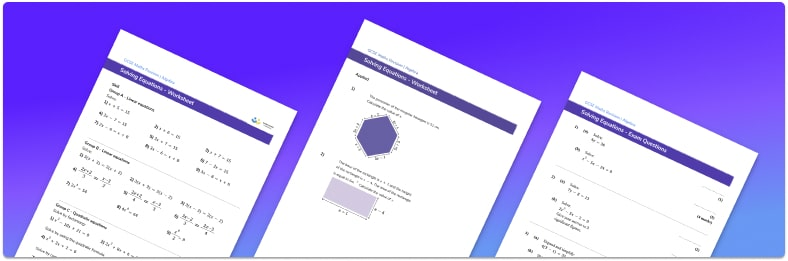# Solving Equations Worksheet• Section 1 of the solving equations worksheet contains 27 skills-based solving equations questions, in 3 groups to support differentiation
• Section 2 contains 2 applied solving equations questions with a mix of worded problems and deeper problem solving questions
• Section 3 contains 3 foundation and higher level GCSE exam style practice questions for the different types of equations, including equations with fractions
• Answers and a mark scheme for all solving equations  questions are provided
• Questions follow variation theory with plenty of opportunities for students to work independently at their own level
• All questions created by fully qualified expert secondary maths teachers
• Suitable for GCSE maths revision for AQA, OCR and Edexcel exam boards

• This field is for validation purposes and should be left unchanged.

You can unsubscribe at any time (each email we send will contain an easy way to unsubscribe). To find out more about how we use your data, see our privacy policy.

### Solving equations at a glance

Solving equations involves rearranging equations to make the unknown the subject in order to work out the solution. To rearrange an equation we use the inverse operation.

There are a variety of different types of equations to solve. The simplest equations are linear one-step equations, two step equations and multi-step equations. Equations can involve fractions and require the use of order of operations to correctly find the unknown variable. Linear equations can also involve brackets which can expanded and they may include an unknown variable on both sides of the equations. Equations involving a square root or indices may be solved in a similar way, by rearranging to make the unknown the subject.

Solving equations also requires solving quadratic equations. Quadratic equations often have two different solutions and can be solved using several different methods. One method involves setting the quadratic expression equal to zero and factorising it into brackets. The solutions can be found by considering each factor being equal to zero. Another method is to solve quadratic equations using the quadratic formula where the coefficients of the different terms are substituted into the quadratic formula. The solutions can be written as surds or decimals. The final method is completing the square which requires careful algebraic manipulation to rearrange the quadratic equations to give two solutions. The solutions are often given in surd form.

Looking forward, students can progress with more solving equations worksheets and to additional algebra worksheets, for example a simplifying expressions worksheet or straight line graphs worksheet.For more teaching and learning support on Algebra our GCSE maths lessons provide step by step support for all GCSE maths concepts.

## Do you have KS4 students who need more focused attention to succeed at GCSE?There will be students in your class who require individual attention to help them succeed in their maths GCSEs. In a class of 30, it’s not always easy to provide.

Help your students feel confident with exam-style questions and the strategies they’ll need to answer them correctly with our dedicated GCSE maths revision programme.

Lessons are selected to provide support where each student needs it most, and specially-trained GCSE maths tutors adapt the pitch and pace of each lesson. This ensures a personalised revision programme that raises grades and boosts confidence.

Find out more